Do you like the article?
Share it with others —
Use new possibilities of MetaTrader 5

#### Similar articles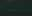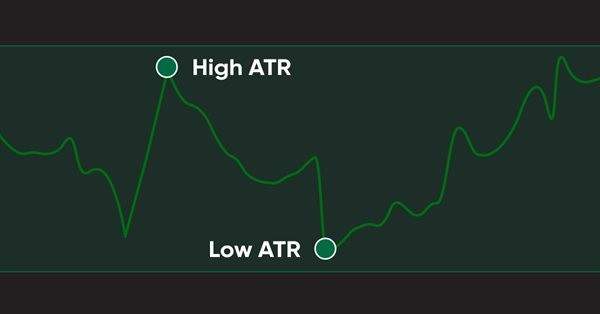# Learn how to design a trading system by ATR10 6924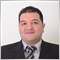### Introduction

In this new article, we will talk about a new concept in trading that we have to measure and know how to use it. This concept is volatility. Many tools can be used to measure the volatility and from these tools, one of the most popular tools is the Average True Range (ATR) technical indicator. When you know how the volatility is going, this can be changing the game like what we will see in this article as it will be one of the important factors or bases to build your decision based on it.

We will go through many topics to understand this ATR indicator very well, as the same as what you may know my approach is to learn and teach the root of things if you read for me other published articles. The following topics cover everything we are going to learn in this article about this amazing tool:

After these topics, I hope that we will understand the ATR indicator deeply and will learn how to use it in a suitable way. We will learn about the Average True Range (ATR) indicator, what it measures, and how it is calculated: we will analyze the formula of its calculation and will apply it on a real example from the Market. This topic will be covered in the "ATR definition" part. We will learn simple strategies that can be used based on the concept of the ATR indicator. I will share simple strategies that can help enhance our understanding — this will be done in the "ATR strategy" part. We will learn to design a blueprint for the mentioned strategies. This is a useful step in the trading system designing process as it visualizes the idea of how the program should work. So, step-by-step blueprints will be provided in the "ATR strategy blueprint" section. Then we will learn how to write the code to design a trading system based on these strategies using the MQL5 programming language. The developed program will then run in the MetaTrader 5 trading platform. This is covered in the "ATR trading system" part.

To write the strategy code in MQL5, we will use MetaQuotes Language Editor (the MetaEditor tool), which is integrated into the MetaTrader 5 trading platform. If you do not have the platform yet, you can download it at https://www.metatrader5.com/en/download.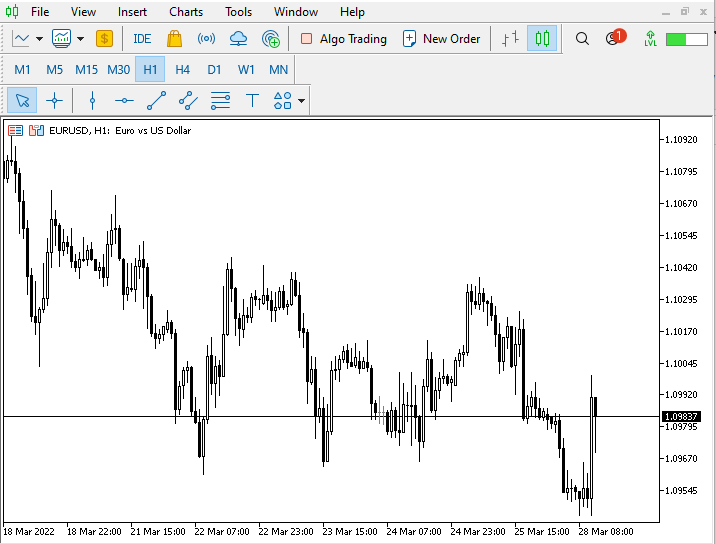From this window, you can open the MetaEditor by pressing the F4 key while the MetaTrader 5 terminal is open. Another way to open it is to click on the IDE button from the MetaTrader 5 terminal interface as shown in the image below: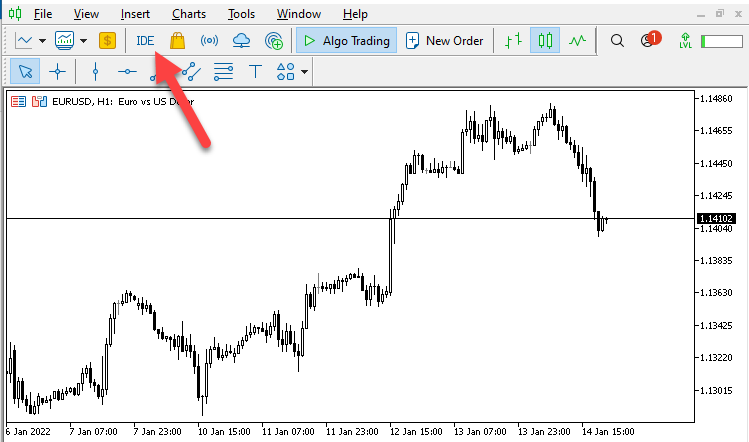Another way to open it is to click on the Tools menu in the MetaTrader 5 terminal --> then choose MetaQuotes Language Editor from the menu as it is shown in the following picture: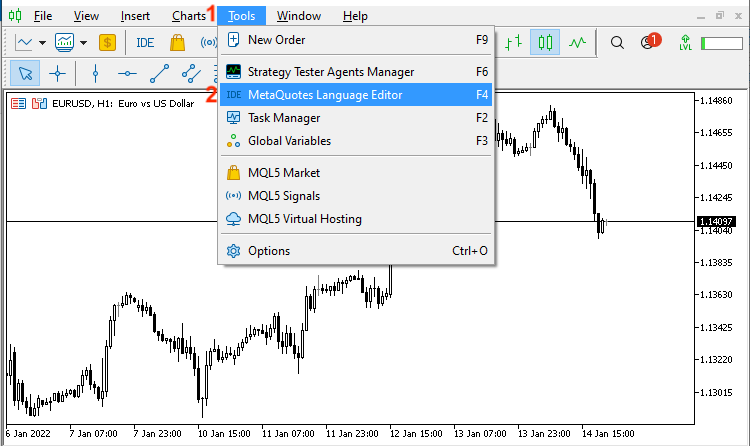This will open the MetaQuotes Language Editor. The following picture shows the interface of it: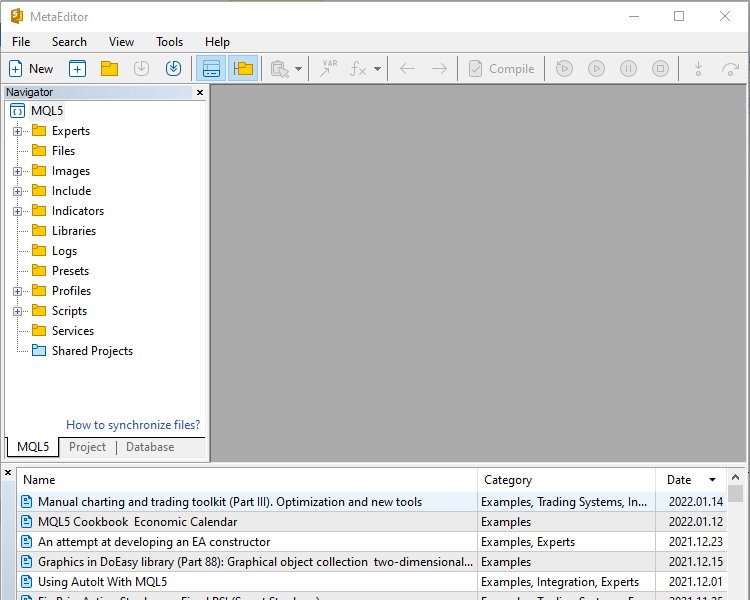Disclaimer: All information is provided 'as is' only for educational purposes and is not prepared for trading purposes or advice. The information does not guarantee any kind of result. If you choose to use these materials on any of your trading accounts, you will do that at your own risk and you will be the only responsible.

### ATR definition

In this topic, we will cover in detail the Average True Range (ATR) indicator: what it measures and how it is calculated, with some examples to deepen our understanding to get more insights about how we can use and benefit from this indicator.

The Average True Range (ATR) technical indicator was developed by Welles Wilder. This ATR indicator measures market volatility by analyzing the range of prices for a specific period. ATR does not provide price or market direction but it provides only the volatility measurement.

Now, here is how the ATR indicator measuring the market volatility is calculated.

First, we need to calculate the true range, which will be the greatest value of the following:

• The difference between current high and low.
• The difference between the current high and the previous close (absolute value).
• The difference between the current low and the previous close (absolute value).

So, here at this step, we need highs, lows, and closing prices data to calculate it. Then, we will calculate the average true range.

Now, let us see an example from the real market to apply what we learned and calculate the average true range (ATR), the following is EURUSD data:

Day High Low Close
Mar 17, 2022 1.1138 1.1008 1.1089
Mar 18, 2022 1.112 1.1003 1.1055
Mar 21, 2022 1.107 1.101 1.1014
Mar 22, 2022 1.1047 1.096 1.1027
Mar 23, 2022 1.1044 1.0963 1.1004
Mar 24, 2022 1.1014 1.0965 1.0996
Mar 25, 2022 1.1039 1.0979 1.0981
Mar 28, 2022 1.1 1.0944 1.0978
Mar 29, 2022 1.1137 1.097 1.1085
Mar 30, 2022 1.1171 1.1083 1.1156
Mar 31, 2022 1.1185 1.1061 1.1065
Apr 01, 2022 1.1077 1.1027 1.1053
Apr 04, 2022 1.1056 1.096 1.097
Apr 05, 2022 1.099 1.0899 1.0903
Apr 06, 2022 1.0939 1.0874 1.0893
Apr 07, 2022 1.0939 1.0864 1.0878
Apr 08, 2022 1.0892 1.0836 1.0876
Apr 11, 2022 1.0951 1.0872 1.0883
Apr 12, 2022 1.0905 1.0821 1.0826
Apr 13, 2022 1.0896 1.0809 1.0885
Apr 14, 2022 1.0925 1.0757 1.0827
Apr 15, 2022 1.0832 1.0796 1.0806
Apr 18, 2022 1.0822 1.0769 1.078
Apr 19, 2022 1.0815 1.0761 1.0786
Apr 20, 2022 1.0868 1.0783 1.085
Apr 21, 2022 1.0937 1.0824 1.0836
Apr 22, 2022 1.0853 1.077 1.0794
Apr 25, 2022 1.0842 1.0697 1.0711
Apr 26, 2022 1.0738 1.0635 1.0643
Apr 27, 2022 1.0655 1.0586 1.0602

Now, to calculate the ATR, we will follow the below steps.

1- Calculate TR, it will be the largest value of the following:

• Current high - current low (the difference between high and low),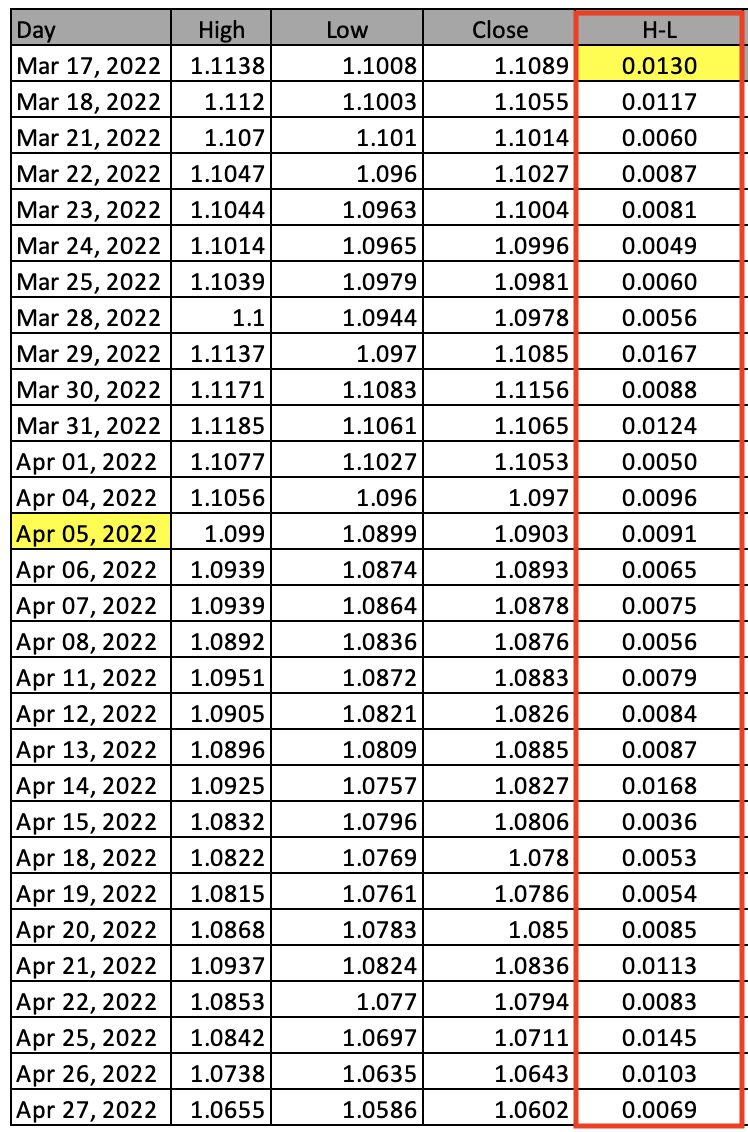• Absolute (current high-previous close),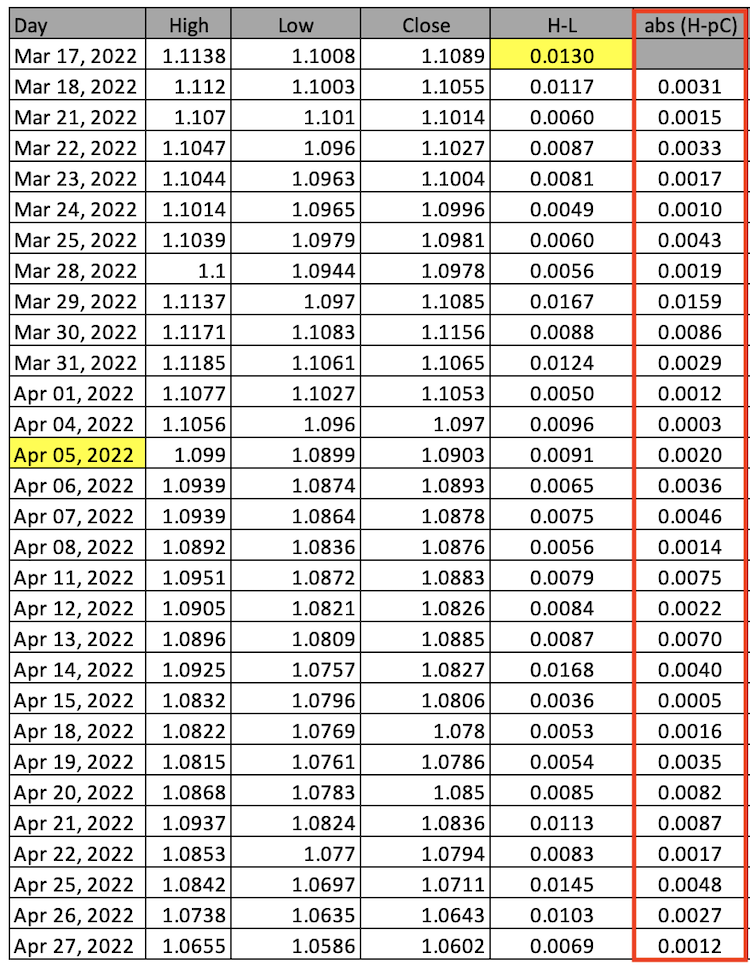• Absolute (current low-previous close),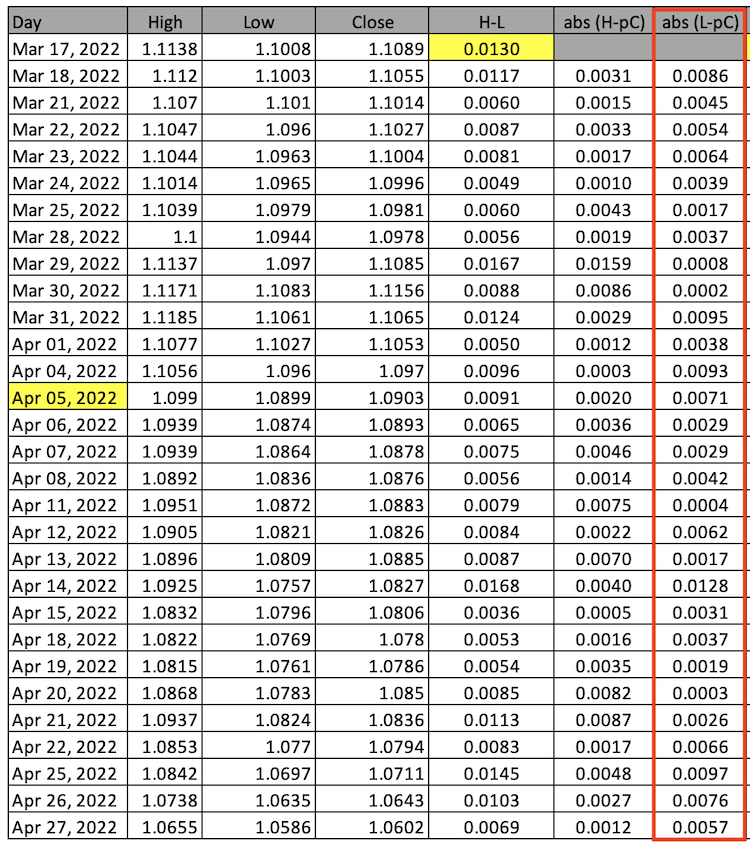According to what we calculated, we will determine the TR that is the largest value from (high-low, abs(high-previous close), abs(low-previous close)),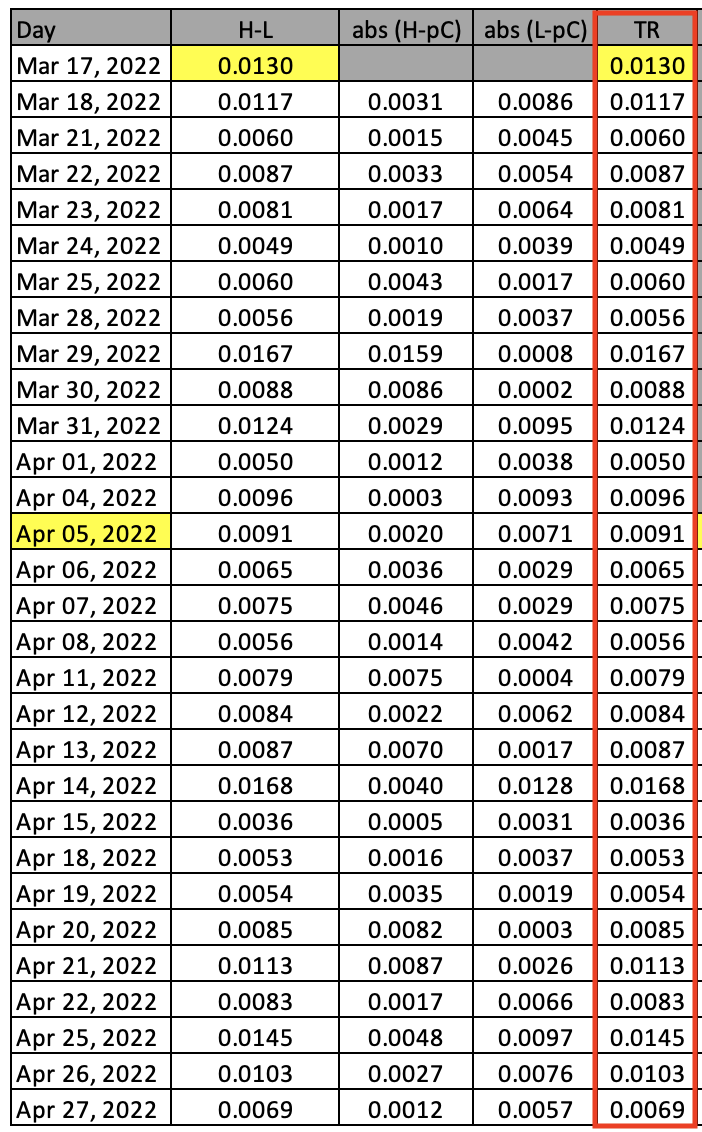2- Calculate the average true range (ATR) for 14 periods,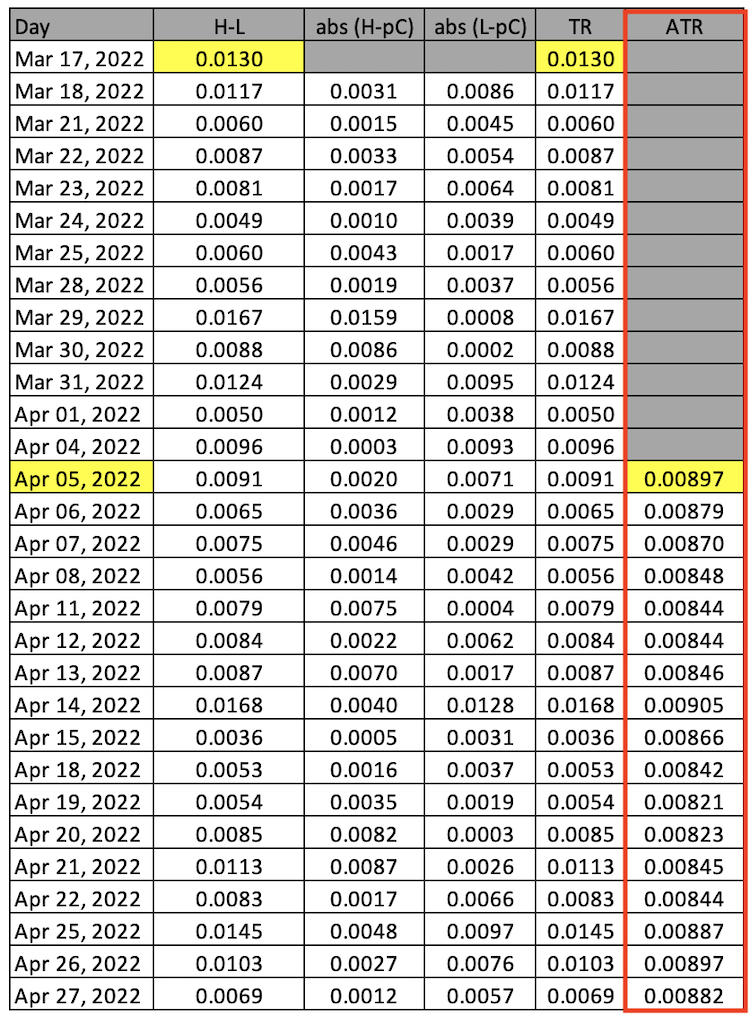By the previous steps, we can calculate the Average True Range (ATR) manually. But nowadays we do not need to do that because we it available in the ready-to-use form in the MetaTrader 5 trading platform. All we need to do is to choose it among other available indicators and the following is how to do that.

While opening the MetaTrader 5 trading terminal, click on insert tab --> indicator --> Oscillators --> Average True Range: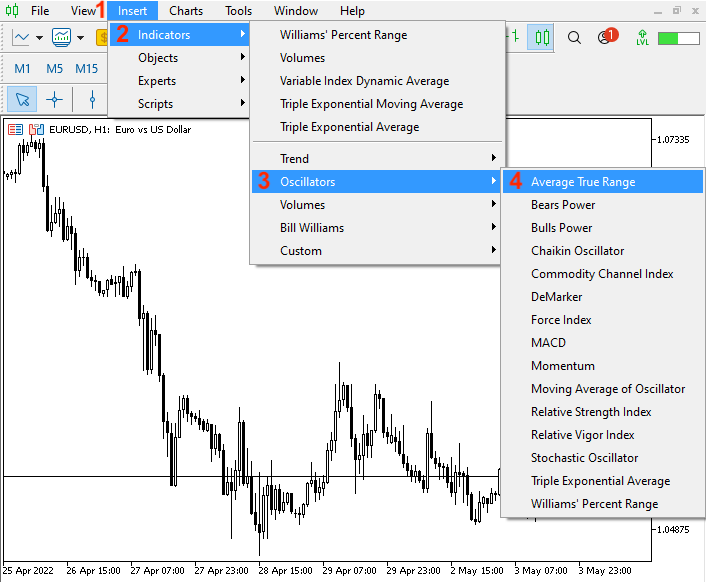After choosing the Average True Range, the following window will be opened for the parameters of the ATR: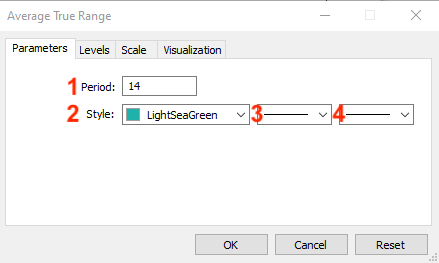1 - the desired period for the indicator.

This value of period can be different from timeframe to another or from one instrument to another as per the volatility of each period or instrument. The longer ATR can be better to generate an accurate indication because it will be a smoother average.

2 - the color of the ATR line.

3 - the ATR line style.

4 - the ATR line thickness.

After setting parameters on the ATR indicator, it will be attached to the chart and will appear as follows: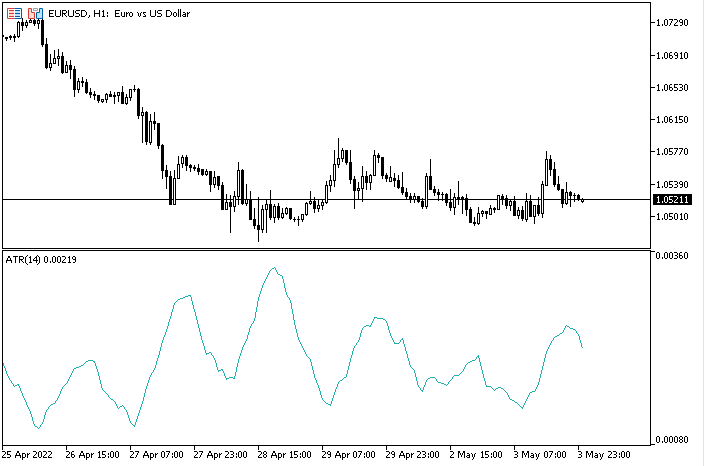As we can see in the previous picture, the lower window includes the 14 periods ATR indicator which is represented by a line oscillating to measure the volatility of EURUSD through the 1H timeframe.

As I mentioned before the ATR measures the volatility. According to this, analyze the values in the ATR window: the lower the ATR value, the lower the volatility of the instrument. And vice versa, the higher the value of the ATR, the higher the volatility of the instrument.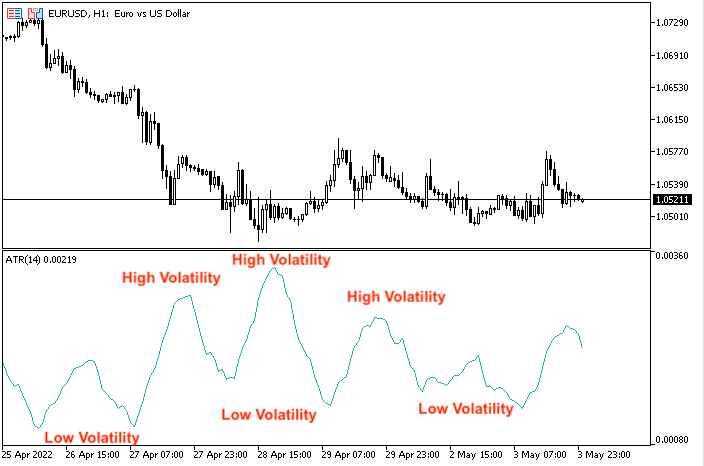So when the ATR records low values, this indicates low volatility, and vice versa, when the ATR indicator records high values, this indicates high volatility.

The ATR indicator is not accurate to generate trading signals as we already know based on its calculation. It considers only the magnitude of range but its beauty is that it is one of the best tools that can help to apply a suitable position size, stop loss, and take profit.

As I mention before, ATR measures only the volatility and it does not provide the trend or the market direction. So, do not get confused if the ATR curve is moving up — this does not mean that the trend is up, and vice versa, if the ATR curve is moving down, this does not mean that the trend is down. If you want to learn about one of most popular tools that is ADX indicator that can be used to determine if there is a trend or no.

Also you can read the trend definition from my previous article.

The following picture is an example that can show that ATR does not provide a trend direction: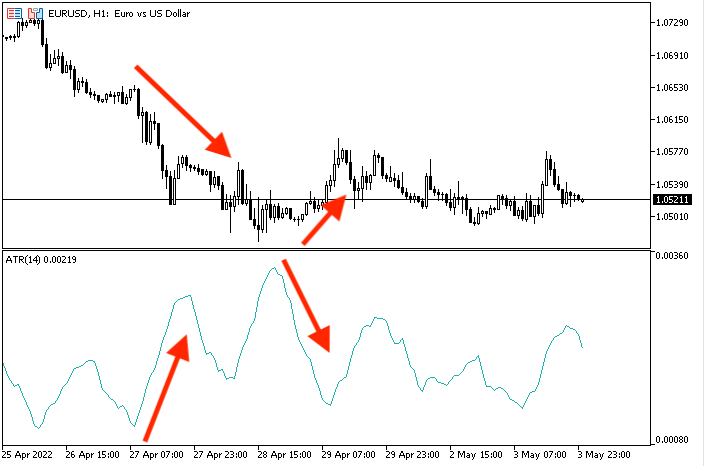As we can see, the indicator is moving up at the same time that prices are moving down and vice versa, the ATR line is moving down at the same time that prices are moving up. This is because ATR is not providing a trend or market direction but it is providing a measurement of the volatility only.

### ATR strategy

In this topic, we will learn simple strategies that can be used with the ATR indicator according to its concept to enhance our trading results. The concept of the ATR indicator is that it measures the volatility of the market only, not the direction of market.

1- Simple ATR System - ATR Strength:

According to this strategy, we will compare between current ATR value and specific values and decide if the ATR is strong or not. It is important here to note that these specific values may differ from one instrument to another or from one period to another. So, we will check the ATR indicator value and determine its strength according to its value. If the current ATR value is greater than 0.0024, this means that ATR is strong and vice versa, if the current ATR value is less than 0.0014, this means that ATR is weak. If the ATR value is between 0.0014 and 0.0024, this means that ATR is neutral and returns the current ATR value only.

• Current ATR Value > 0.0024 = ATR is strong
• Current ATR Value < 0.0014 = ATR is weak
• Current ATR Value > 0.0014 and < 0.0024 = current ATR value

2 - Simple ATR System - ATR Movement:

According to this strategy, we will compare the current ATR value with the previous ATR value and then decide on the ATR movement, or if the ATR line moves up and that will happen when the current ATR value is greater than the previous ATR value or it moves down and that will happen when current ATR value is less than previous ATR value.

• Current ATR value > previous ATR value =  ATR is UP
• Current ATR value < previous ATR value = ATR is down

3 - Simple ATR System - SL and TP levels:

According to this strategy, we need to use the ATR values to determine a dynamic stop loss and take profit level based on the current ATR value and specific calculation for stop loss and take profit in both cases the buy position and sell position.

In the case of the buy position, we need to see on the chart:

• A new line with the current bid price.
• A new line with the current ATR value.
• A new line with the stop loss value according to a specific calculation for the buy position.
• A new line with the take profit value according to a specific calculation for the buy position.

In the case of the sell position, we need to see on the chart:

• A new line with the current bid price.
• A new line with the current ATR value.
• A new line with the stop loss value according to a specific calculation for the sell position.
• A new line with the take profit value according to a specific calculation for the sell position.

We can find that there are other advanced strategies that can be used by the ATR indicator but here we need to mention only some simple strategies that can help us to understand the ATR indicator deeply to be able to use it in a proper way that can enhance our trading results.

### ATR Strategy blueprint

In this part, we learn step-by-step what we need in order to create a trading system for the three mentioned strategies through a blueprint for every strategy. First and before going through designing or creating the three mentioned strategies, we will start with a blueprint for needed steps to create a simple program or Expert Advisor that can show us a comment with the current ATR value to help us to design our mentioned strategies and the following is a picture that represents the blueprint for that: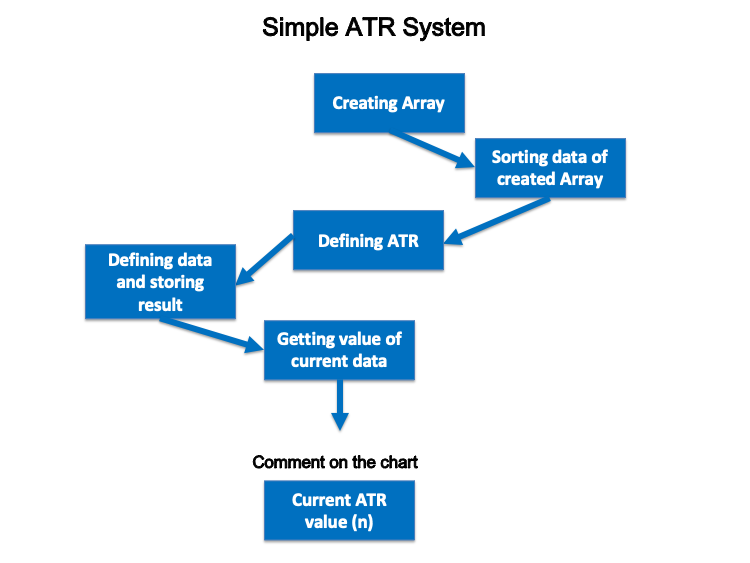1- Simple ATR System - ATR Strength:

• Current ATR Value > 0.0024 = ATR is strong
• Current ATR Value < 0.0014 = ATR is weak
• Current ATR Value > 0.0014 and < 0.0024 = current ATR value

So, we want the program to check the current ATR value with specific values and decide if the current ATR value is greater than 0.0024 - this would mean that ATR is strong. If it is not greater than 0.0024, we need the program to check if the ATR is less than 0.0014 - this would mean that the ATR is weak. In case it is not less than is 0.0014 value and the program finds it between 0.0014 and 0.0024, then we want the program to show us with the current ATR value only on the chart.

The following is a blueprint of this ATR strength strategy: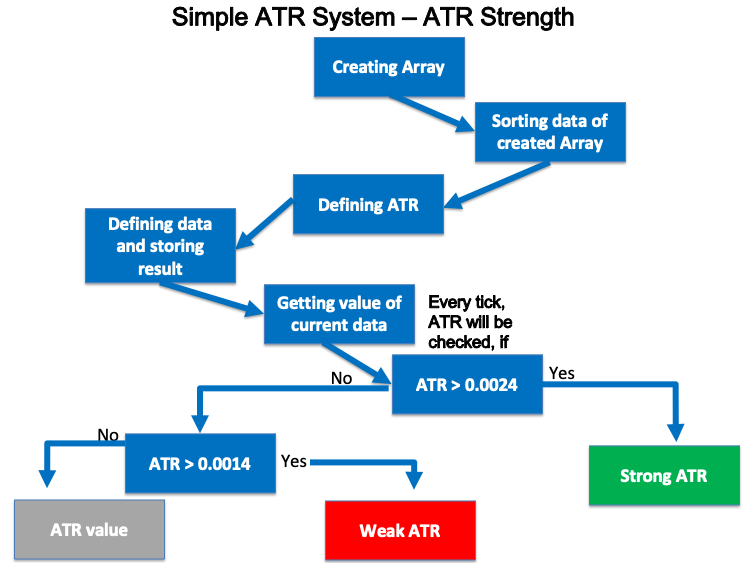2 - Simple ATR System - ATR Movement:

• Current ATR value > previous ATR value =  ATR is UP
• Current ATR value < previous ATR value = ATR is down

So, we need the Expert Advisor to check the current ATR value and the previous ATR value then decide, if the current ATR value is greater than the previous ATR value, this means that the ATR is up and if the current ATR value is less than the previous ATR value, this means that the ATR is down, or it gives nothing.

The following is a blueprint of this ATR movement strategy: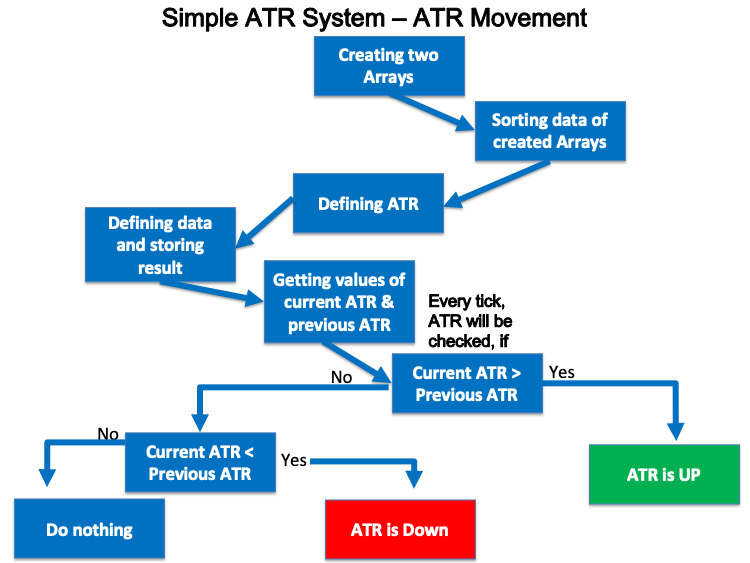3 - Simple ATR System - SL and TP levels:

It needed to appear a comment on the chart in both cases,

The buy position and the sell position:

• A new line with the current bid price.
• A new line with the current ATR value.
• A new line with the stop loss value.
• A new line with the take profit value.

And the following is a blueprint of this ATR SL and TP levels strategy: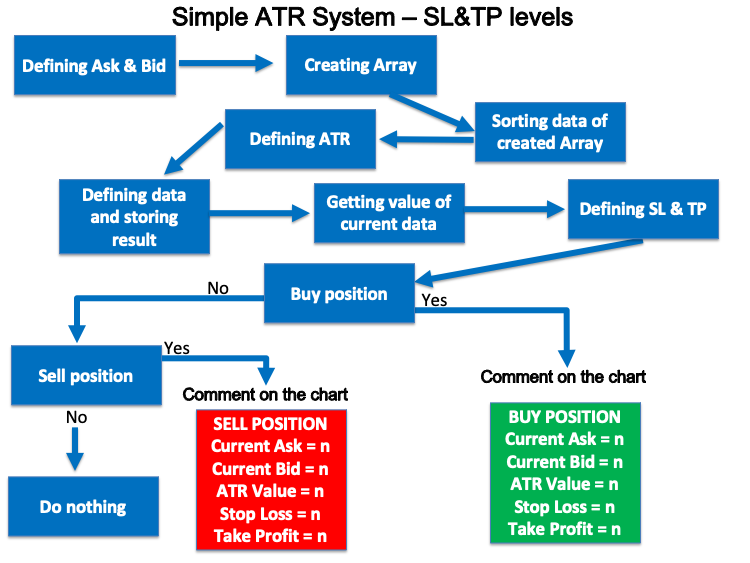In this interesting topic, we will learn to write the code of every mentioned strategy to create a trading system based on each one of them be to executed easily, smoothly, and accurately. Now, we will write a code for an Expert Advisor to show us the ATR value on the chart automatically.

The following is how we can write the code of this Expert Advisor of the Simple ATR System:

• Creating price array by using the "double" function:
`double PriceArray[];`
• Sorting data by using "ArraySetAsSeries" function:
`ArraySetAsSeries(PriceArray,true);`
• Defining the ATR by using the iATR function and integer "int" value for returned value:
`int ATRDef=iATR(_Symbol,_Period,14);`
• Defining data and storing results by using "the CopyBuffer" function:
`CopyBuffer(ATRDef,0,0,3,PriceArray);`
• Getting the value of current data by returning a double value by using the "NormalizeDouble" function:
`double ATRValue=NormalizeDouble(PriceArray,5);`
• Getting a comment on the chart with the ATR value:
`Comment("ATR Value = ",ATRValue);`

The following is the complete code if you want to copy and paste it easily, or to see it in one block:

```//+------------------------------------------------------------------+
//|                                                   Simple ATR.mq5 |
//|                                  Copyright 2022, MetaQuotes Ltd. |
//|                                             https://www.mql5.com |
//+------------------------------------------------------------------+
#property version   "1.00"
//+------------------------------------------------------------------+
void OnTick()
{
//creating price array
double PriceArray[];

//Sorting data
ArraySetAsSeries(PriceArray,true);

//define ATR
int ATRDef=iATR(_Symbol,_Period,14);

//define data and store result
CopyBuffer(ATRDef,0,0,3,PriceArray);

//get value of current data
double ATRValue=NormalizeDouble(PriceArray,5);

//comment on the chart
Comment("ATR Value = ",ATRValue);
}
//+------------------------------------------------------------------+```

After writing this Expert Advisor , if we want to execute this program, we can find it in the navigator the same as the following picture: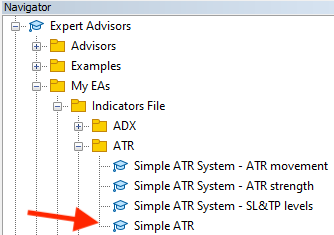By double-clicking or dragging and dropping this file on the chart, the following window will be opened,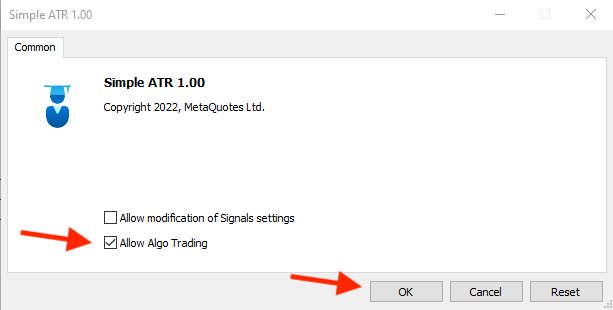After clicking "OK", we can find that the Simple ATR Expert Advisor is attached to the chart the same as the following picture: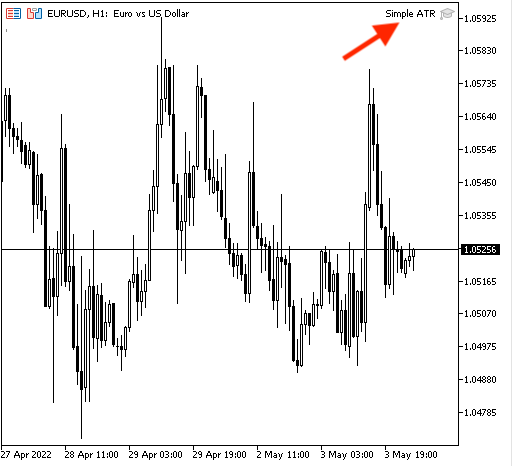And we can find its result according to the program the same as the following example from testing: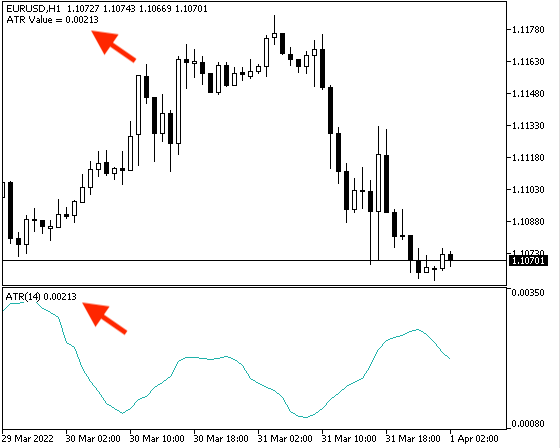Now, we will create a trading system for each mentioned strategy and it will be the same as the below,

1- Simple ATR System - ATR Strength:

• Current ATR Value > 0.0024 = ATR is strong
• Current ATR Value < 0.0014 = ATR is weak
• Current ATR Value > 0.0014 and < 0.0024 = current ATR value

The following is how we can write the code of this Expert Advisor of Simple ATR System - ATR Strength:

```//+------------------------------------------------------------------+
//|                             Simple ATR System - ATR strength.mq5 |
//|                                  Copyright 2022, MetaQuotes Ltd. |
//|                                             https://www.mql5.com |
//+------------------------------------------------------------------+
#property version   "1.00"
//+------------------------------------------------------------------+
void OnTick()
{
//creating price array
double PriceArray[];

//Sorting data
ArraySetAsSeries(PriceArray,true);

//define ATR
int ATRDef=iATR(_Symbol,_Period,14);

//define data and store result
CopyBuffer(ATRDef,0,0,3,PriceArray);

//get value of current data
double ATRValue=NormalizeDouble(PriceArray,5);

//comment on the chart with ATR strength as per its value
if(ATRValue>0.0024)
Comment("Strong ATR","\n","ATR Value = ",ATRValue);

if(ATRValue<0.0014)
Comment("Weak ATR","\n","ATR Value = ",ATRValue);

if((ATRValue>0.0014)&&(ATRValue<0.0024))
Comment("ATR Value = ",ATRValue);
}
//+------------------------------------------------------------------+```

Differences in this code are:

• Commenting based on comparing current ATR with a specific value, if current ATR value > 0.0024:
```   if(ATRValue>0.0024)
Comment("Strong ATR","\n","ATR Value = ",ATRValue);```
• If current ATR < 0.0014
```   if(ATRValue<0.0014)
Comment("Weak ATR","\n","ATR Value = ",ATRValue);```
• If current ATR value > 0.0014 and current ATR < 0.0024
```   if((ATRValue>0.0014)&&(ATRValue<0.0024))
Comment("ATR Value = ",ATRValue);```

After that, we can find this Expert Advisor in the navigator window to execute it after compiling it: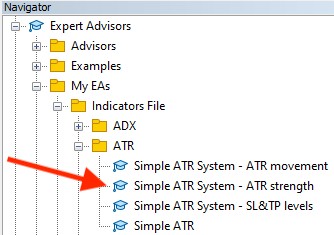After opening the file by double-clicking or dragging and dropping it on the chart, we can see the window the same as the following: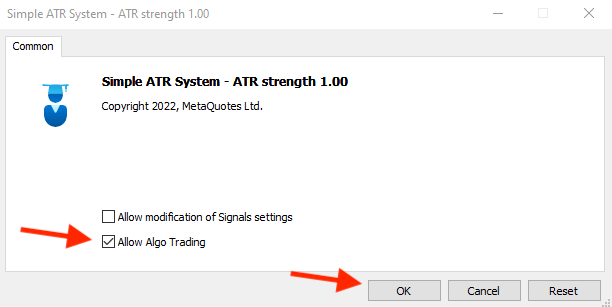After pressing "OK", we can find the Expert Advisor attached to the chart the same as the following picture:And the following is an example of the generated signals based on this strategy for testing:

As we can see in the following chart it shows a comment with Strong ATR and current ATR value in a new line: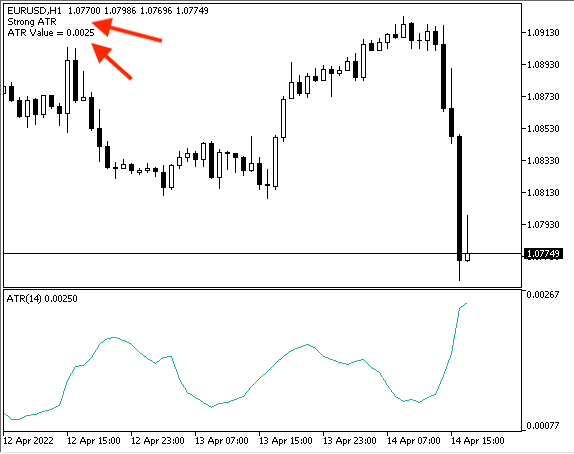As we can see the following chart shows a comment with weak ATR and current ATR value in a new line: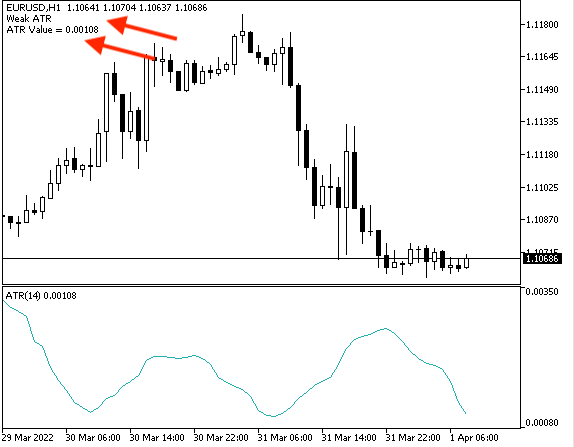As we can see the following chart shows a comment with current ATR value only because it neutral as per determined values: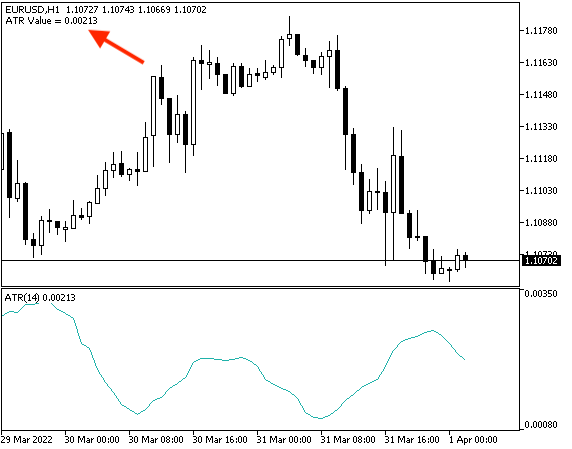2 - Simple ATR System - ATR Movement:

• Current ATR value > previous ATR value =  ATR is UP
• Current ATR value < previous ATR value = ATR is down

The following is how we can write code of this Expert Advisor of Simple ATR System - ATR Movement:

```//+------------------------------------------------------------------+
//|                             Simple ATR System - ATR movement.mq5 |
//|                                  Copyright 2022, MetaQuotes Ltd. |
//|                                             https://www.mql5.com |
//+------------------------------------------------------------------+
#property version   "1.00"
//+------------------------------------------------------------------+
void OnTick()
{
//creating arrays
double PriceArray0[];
double PriceArray1[];

//sort price array from current data
ArraySetAsSeries(PriceArray0,true);
ArraySetAsSeries(PriceArray1,true);

//define ATR
int ATRDef=iATR(_Symbol,_Period,14);

//define data and store result
CopyBuffer(ATRDef,0,0,3,PriceArray0);
CopyBuffer(ATRDef,0,0,3,PriceArray1);

//get value of current data
double ATRValue=NormalizeDouble(PriceArray0,5);
double PreATRValue=NormalizeDouble(PriceArray1,5);

if(ATRValue>PreATRValue)
Comment("ATR is UP","\n","ATR Value = ",ATRValue,"\n","ATR Previous Value = ",PreATRValue);

if(ATRValue<PreATRValue)
Comment("ATR is Down","\n","ATR Value = ",ATRValue,"\n","ATR Previous Value = ",PreATRValue);

}
//+------------------------------------------------------------------+```

Differences in this code are:

• Creating two price arrays (current ATR value(PriceArray0), previous ATR value(PriceArray1)):
```   double PriceArray0[];
double PriceArray1[];```
• Sorting two price arrays from current data:
```   ArraySetAsSeries(PriceArray0,true);
ArraySetAsSeries(PriceArray1,true);```
• Defining data and storing result for two arrays:
```   CopyBuffer(ATRDef,0,0,3,PriceArray0);
CopyBuffer(ATRDef,0,0,3,PriceArray1);```
• Getting value of current data for two value (current and previous ATR):
```   double ATRValue=NormalizeDouble(PriceArray0,5);
double PreATRValue=NormalizeDouble(PriceArray1,5);```
• Commenting based on comparing current ATR with previous ATR value,
• If current ATR value > Previous ATR value:
```   if(ATRValue>PreATRValue)
Comment("ATR is UP","\n","ATR Value = ",ATRValue,"\n","ATR Previous Value = ",PreATRValue);```
• If current ATR value < previous ATR value:
```   if(ATRValue<PreATRValue)
Comment("ATR is Down","\n","ATR Value = ",ATRValue,"\n","ATR Previous Value = ",PreATRValue);```
After Compiling, we can find this Expert Advisor in the navigator window to execute it: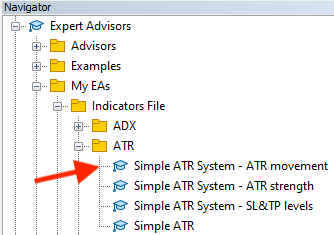y double-clicking or dragging and dropping the file on the chart, we can see the window the same as the following: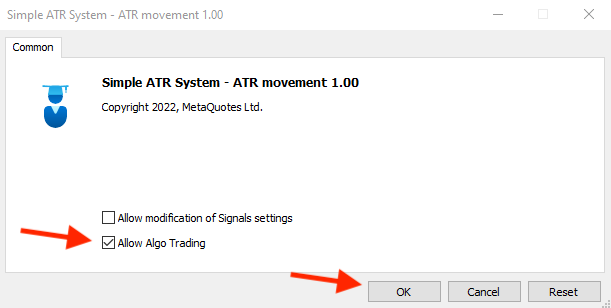After enabling the "Allow Algo Trading" pressing "OK" option, we can find the Expert Advisor attached to the chart the same as the following picture: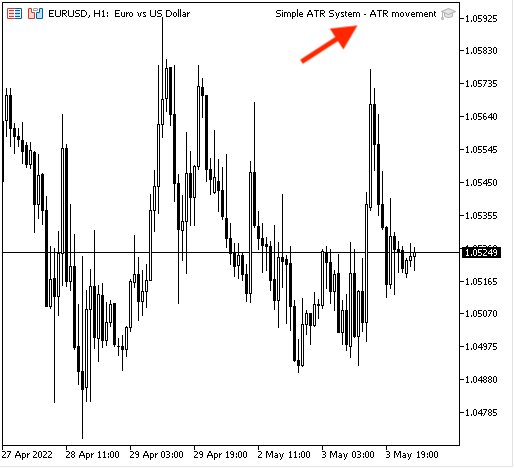And the following is an example of the generated signals based on this strategy for testing,

In case of ATR is up: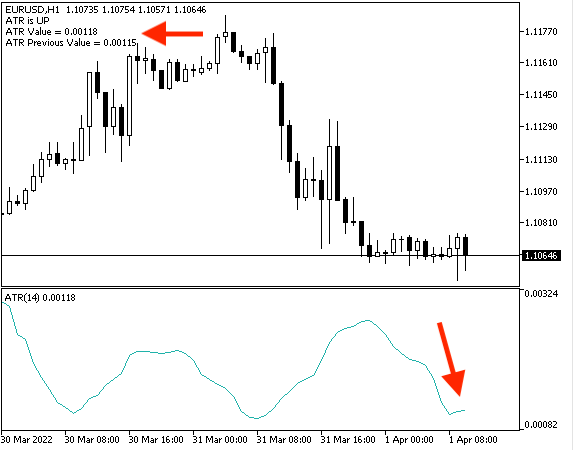In case of ATR is down: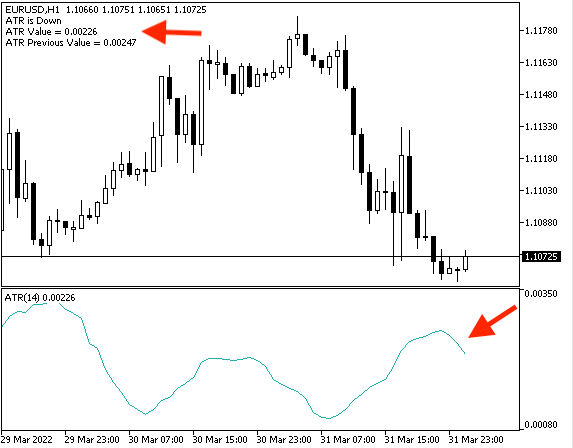3 - Simple ATR System - SL and TP levels:

In the case of the buy position, we need to see on the chart:

• A new line with the current bid price.
• A new line with the current ATR value.
• A new line with the stop loss value for the buy position.
• A new line with the take profit value for the buy position.

In the case of the sell position, we need to see on the chart:

• A new line with the current bid price.
• A new line with the current ATR value.
• A new line with the stop loss for the sell position.
• A new line with the take profit for the sell position.

The following is how we can write code of this Expert Advisor of Simple ATR System - SL and TP levels:

```//+------------------------------------------------------------------+
//|                             Simple ATR System - SL&TP levels.mq5 |
//|                                  Copyright 2022, MetaQuotes Ltd. |
//|                                             https://www.mql5.com |
//+------------------------------------------------------------------+
#property version   "1.00"
//+------------------------------------------------------------------+
void OnTick()
{
double Bid=NormalizeDouble(SymbolInfoDouble(_Symbol,SYMBOL_BID),_Digits);

//creating price array
double PriceArray[];

//Sorting data
ArraySetAsSeries(PriceArray,true);

//define ATR
int ATRDef=iATR(_Symbol,_Period,14);

//define data and store result
CopyBuffer(ATRDef,0,0,3,PriceArray);

//get value of current data
double ATRValue=NormalizeDouble(PriceArray,5);

//Calculate SL&TP for sell position
double TakeProfitSell=Bid-((ATRValue*2)*2);

//comment on the chart
"\n","Stop Loss = ",StopLossSell,"\n","Take Profit = ",TakeProfitSell);
}
//+------------------------------------------------------------------+```

Differences here are:

• Defining Ask and Bid prices to calculate stop loss and take profit:
```   double Ask=NormalizeDouble(SymbolInfoDouble(_Symbol,SYMBOL_ASK),_Digits);
double Bid=NormalizeDouble(SymbolInfoDouble(_Symbol,SYMBOL_BID),_Digits);```
• Calculating the stop loss and take profit for both buy position and sell position:
```   //Calculate SL&TP for buy position

//Calculate SL&TP for sell position
double TakeProfitSell=Bid-((ATRValue*2)*2);```
• Comment based on the strategy for both the buy position and sell position:
• A new line with the current bid price.
• A new line with the current ATR value.
• A new line with the stop loss value.
• A new line with the take profit value.
• ```   Comment("BUY POSITION","\n","Current Ask = ",Ask,"\n","Current Bid = ",Bid,"\n","ATR Value = ",ATRValue,
"\n","Stop Loss = ",StopLossSell,"\n","Take Profit = ",TakeProfitSell);```

After we compile the code, we can find this Expert Advisor in the navigator window to execute it: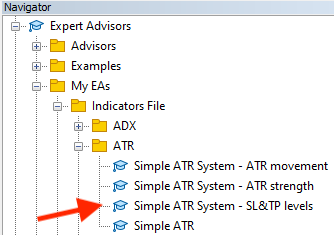After opening the file by double-clicking or dragging and dropping on the chart, the following window will be opened: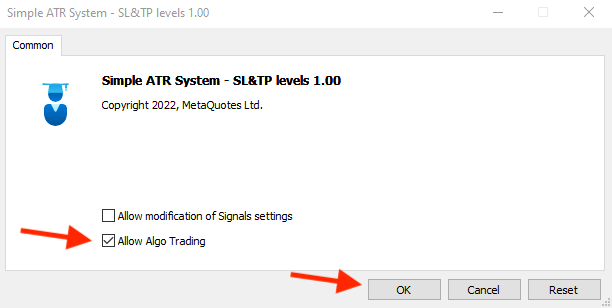After pressing "OK", we can find the Expert Advisor attached to the chart the same as the following picture: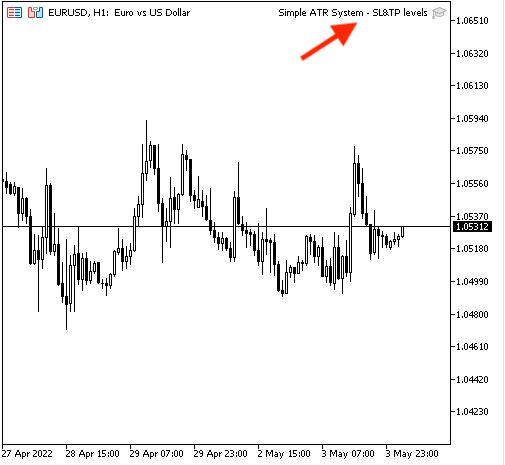And the following is an example of the generated signals based on this strategy for testing: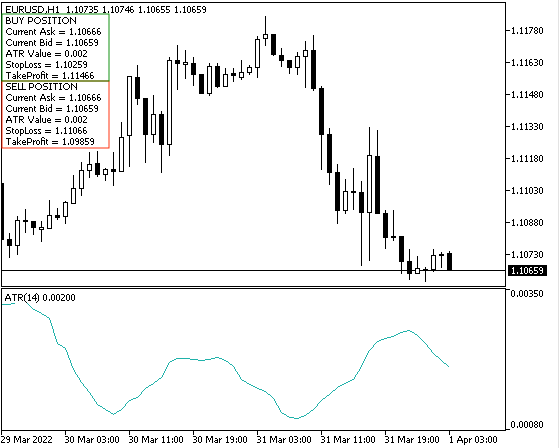### Conclusion

Now, it is supposed that you understand the Average True Range in detail as we learned what is the ATR, what it measures and the concept behind it, how we can calculate it manually, and applied that to a real example from the market and that was through the topic of ATR definition. Then, we learned some simple strategies that can be used in our favor based on the concept of the average true range the same as we learned, we knew the ATR strength system, ATR movement, and Simple ATR - SL & TP levels strategies and this what we learned in the topic of ATR strategy, we learned and designed a blueprint for each strategy to help us to design a trading system for every strategy to work on MetaTrader 5 trading platform through the topic of ATR strategy blueprint topic, then we learn how to write the of Expert Advisor for each strategy by MetaQuotes Languages (MQL5) to execute them on MetaTrader 5 platform through ATR trading system topic.

After understanding and practicing the previous topics, you have to be able to use the average true range (ATR) according to the concept behind it and you have to be able to read the indicator to determine the volatility of the instrument through an automated trading system, especially after applying what you learned by yourself because practicing is important to master anything.

It is important also to confirm that you must test any new strategy or tool before using it on your real account as it may be not useful or profitable for your trading even if it works with someone else as it may be not suitable for your character or your trading plan and this is normal. So, you have to make sure that it is useful and profitable for you by testing first.

#### Other articles by this author

Last comments | Go to discussion (4)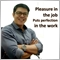| 13 Jun 2022 at 12:47
MetaQuotes:

New article Learn how to design a trading system by ATR has been published:

Author: Mohamed Abdelmaaboud

Very informative and useful article. Thank you for taking the time to write it down.

Just to share, I changed the value a little (array index), at the time of data collection.

Originally:

```   //get value of current data
double ATRValue=NormalizeDouble(PriceArray0,5);
double PreATRValue=NormalizeDouble(PriceArray1,5);```

Become:

```   //get value of current data
double ATRValue=NormalizeDouble(PriceArray0,5);
double PreATRValue=NormalizeDouble(PriceArray1,5);```

And the result is quite good:| 13 Jun 2022 at 13:27
Nino Guevara Ruwano #:

Very informative and useful article. Thank you for taking the time to write it down.

Just to share, I changed the value a little (array index), at the time of data collection.

Originally:

Become:

And the result is quite good:

Thanks for your comment and contribution.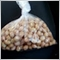| 18 Jun 2022 at 04:32
Thank you very much Mohamed| 18 Jun 2022 at 14:40
Sibusiso Steven Mathebula #:
Thank you very much Mohamed

You are welcome, thanks for your comment.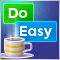Graphics in DoEasy library (Part 98): Moving pivot points of extended standard graphical objects
In the article, I continue the development of extended standard graphical objects and create the functionality for moving pivot points of composite graphical objects using the control points for managing the coordinates of the graphical object pivot points.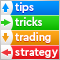Tips from a professional programmer (Part III): Logging. Connecting to the Seq log collection and analysis system
Implementation of the Logger class for unifying and structuring messages which are printed to the Experts log. Connection to the Seq log collection and analysis system. Monitoring log messages online.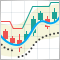Multiple indicators on one chart (Part 04): Advancing to an Expert Advisor
In my previous articles, I have explained how to create an indicator with multiple subwindows, which becomes interesting when using custom indicators. This time we will see how to add multiple windows to an Expert Advisor.Multiple indicators on one chart (Part 03): Developing definitions for users
Today we will update the functionality of the indicator system for the first time. In the previous article within the "Multiple indicators on one chart" we considered the basic code which allows using more than one indicator in a chart subwindow. But what was presented was just the starting base of a much larger system.